##### Trigonometry Workbook For DummiesFunctions with more than one rule (called piece-wise functions) are broken into pieces, depending on the input. Although a piece-wise function has more than one function, each function is defined only on a specific interval. Basically, which piece of the function used depends on the input, and the graph of the function sometimes looks like it’s literally been broken into pieces.

For example, the following represents a piece-wise function: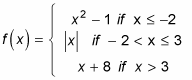This function is broken into three pieces, depending on the domain values for each piece:

• The first piece is the quadratic function f(x) = x2 – 1 and is applied only on the interval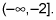As long as the input for this function is less than or equal to –2, apply the first piece (the top line).

• The second piece is the absolute-value function f(x) = |x| and is applied only on the interval (–2, 3].

• The third piece is the linear function f(x) = x + 8 and is applied only on the interval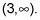To graph this example function, follow these steps:

1. Lightly sketch out the graph y=x2-1 and darken all the values to the left of x = –2.

Because of the interval of the quadratic function of the first piece, you darken all points to the left of –2. And because x = –2 is included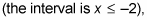the circle at x = –2 is filled in.

2. Between –2 and 3, the graph moves to the second function of the equation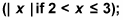3. sketch the absolute-value graph, but pay attention only to the x values between –2 and 3.

You don’t include –2 (open circle), but the 3 is included (closed circle).

4. For x values bigger than 3, the graph follows the third function of the equation: x + 8 if x > 3.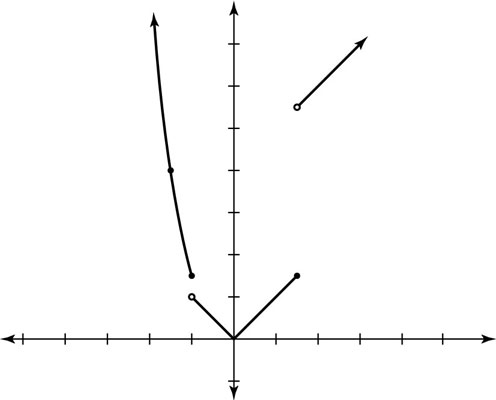You sketch this linear function (where b = 8 with a slope of 1), but only to the right of x = 3 (that point is an open circle since 3 is not included). The finished product is shown in the preceding figure.

Notice that you can’t draw the graph of this piece-wise function without lifting your pencil from the paper. Therefore, this is a discontinuous function.